Скачать презентацию 4 -6 CONGRUENCE IN RIGHT TRIANGLES To prove

f3a6bb00a6cebdd1aef807dffc4f0e4e.ppt

• Количество слайдов: 17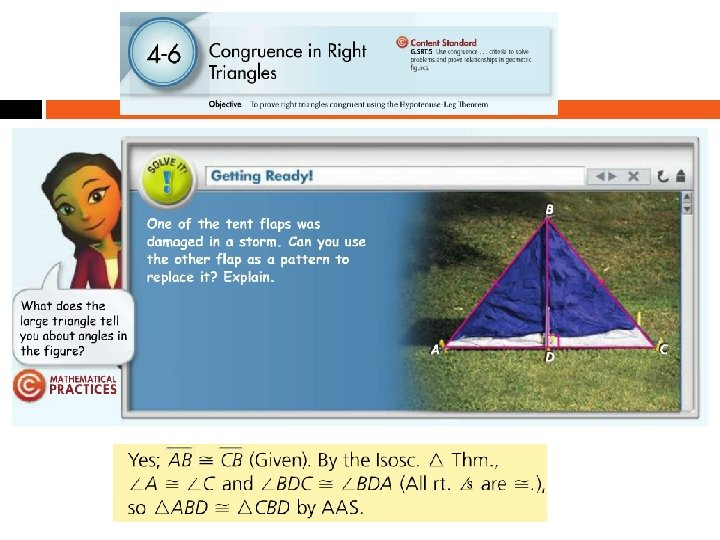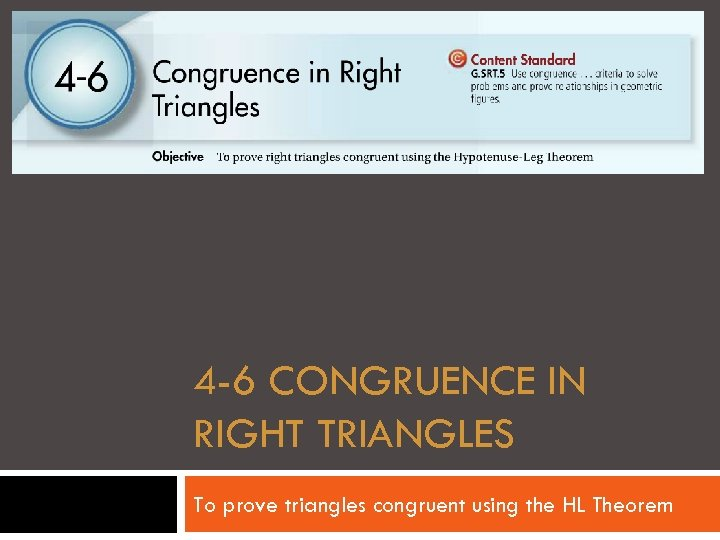4 -6 CONGRUENCE IN RIGHT TRIANGLES To prove triangles congruent using the HL Theorem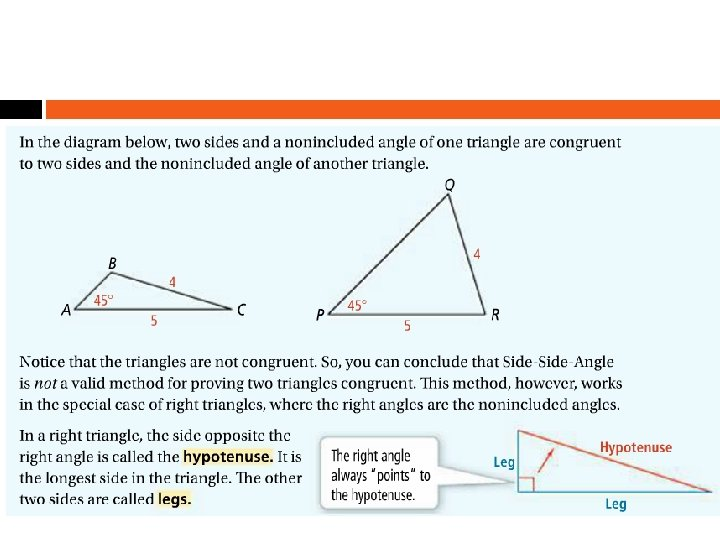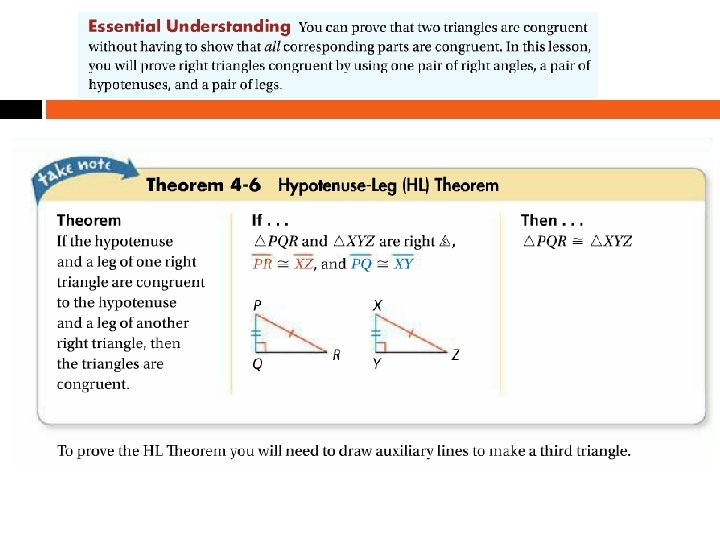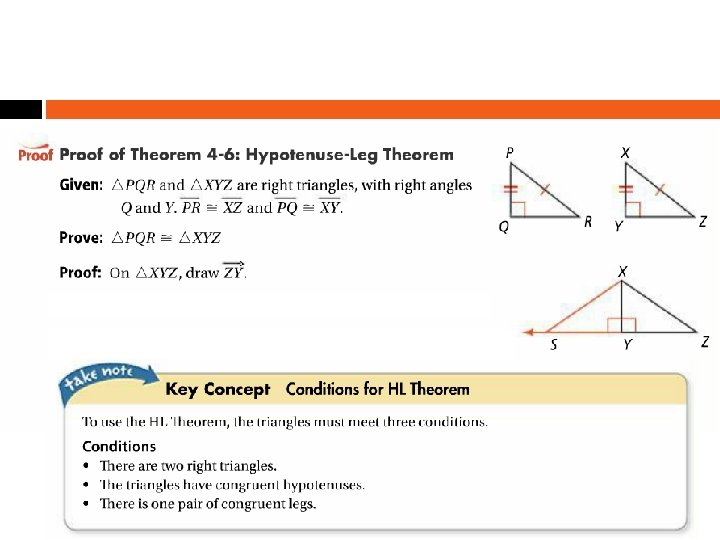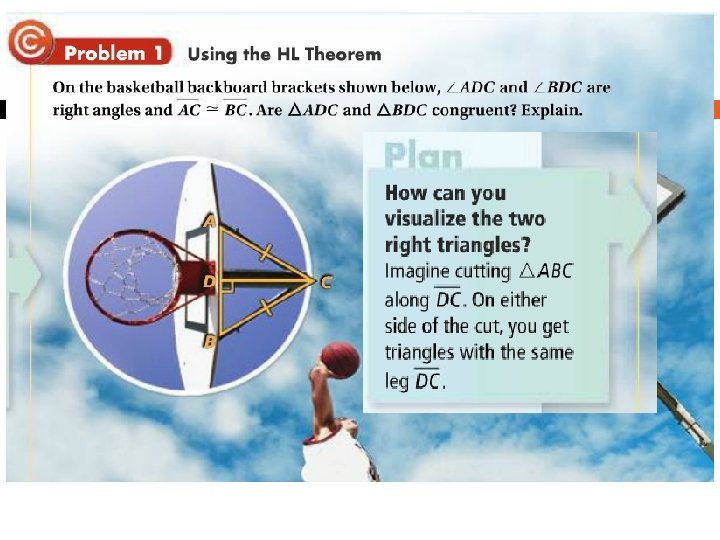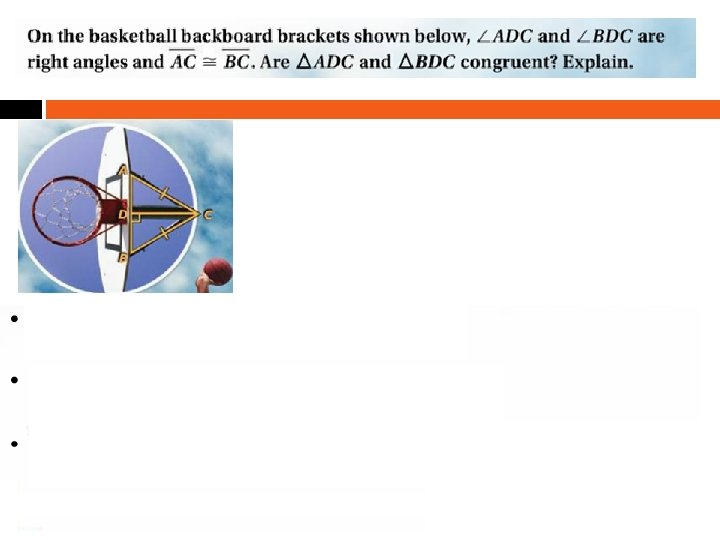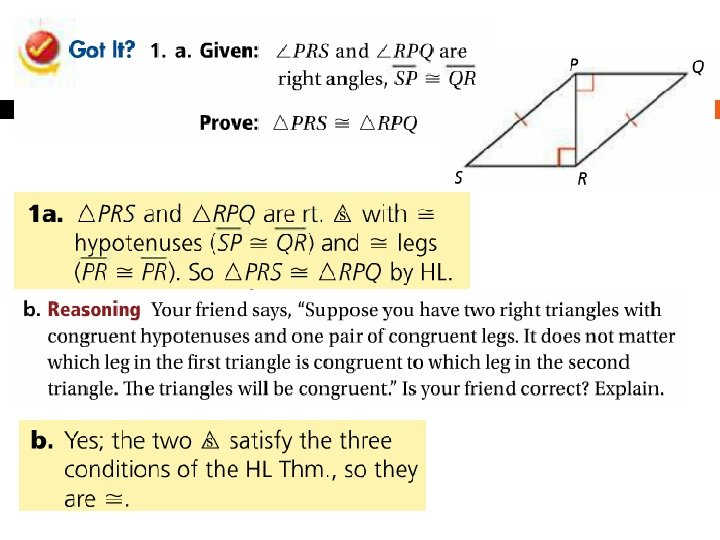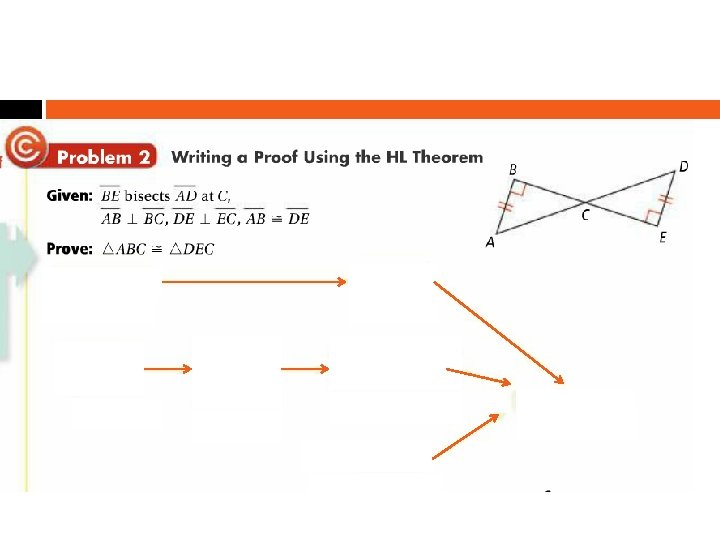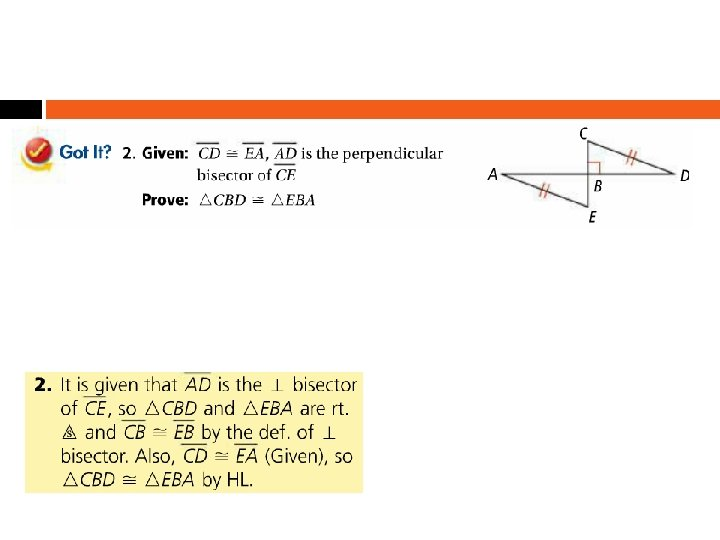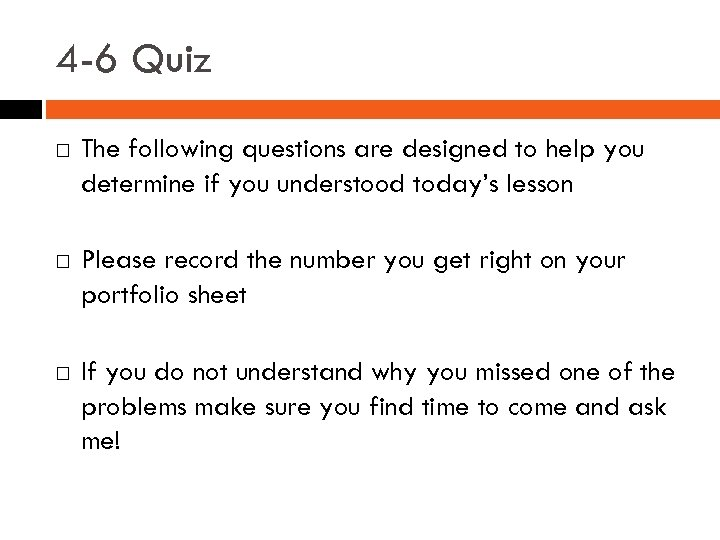4 -6 Quiz The following questions are designed to help you determine if you understood today’s lesson Please record the number you get right on your portfolio sheet If you do not understand why you missed one of the problems make sure you find time to come and ask me!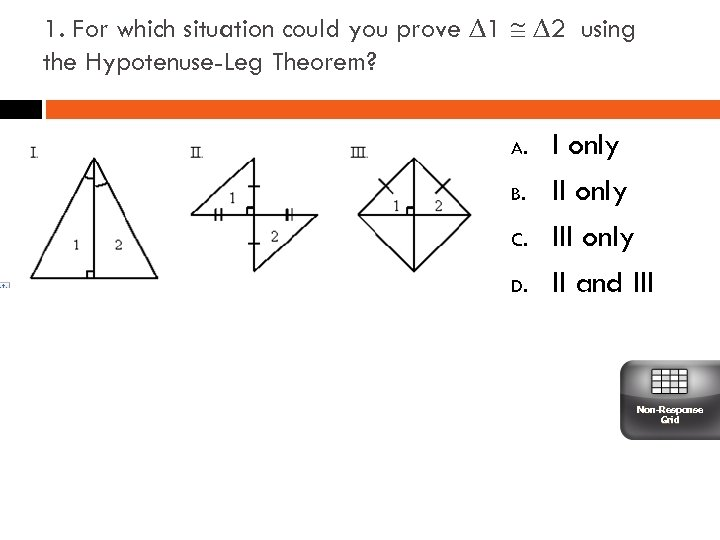1. For which situation could you prove ∆1 ∆2 using the Hypotenuse-Leg Theorem? A. B. C. D. I only III only II and III Non-Response Grid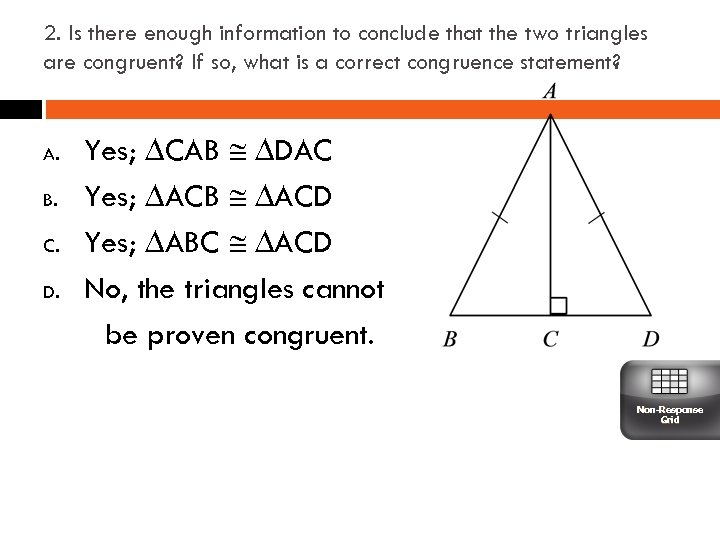2. Is there enough information to conclude that the two triangles are congruent? If so, what is a correct congruence statement? A. B. C. D. Yes; ∆CAB ∆DAC Yes; ∆ACB ∆ACD Yes; ∆ABC ∆ACD No, the triangles cannot be proven congruent. Non-Response Grid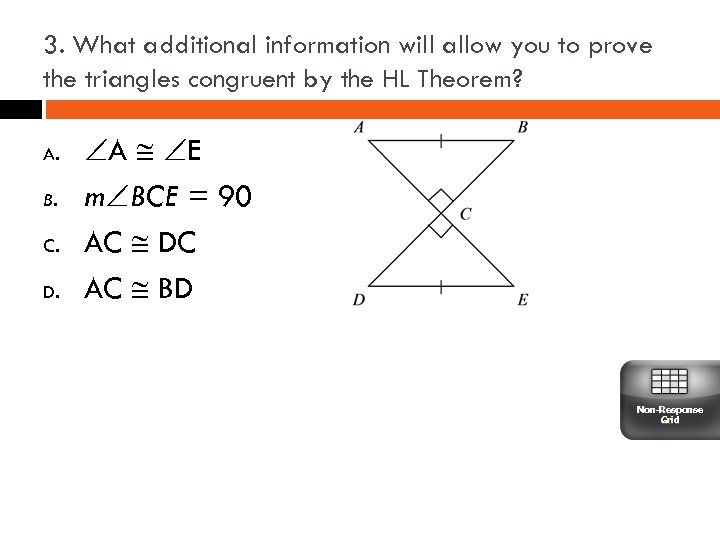3. What additional information will allow you to prove the triangles congruent by the HL Theorem? A. B. C. D. A E m BCE = 90 AC DC AC BD Non-Response Grid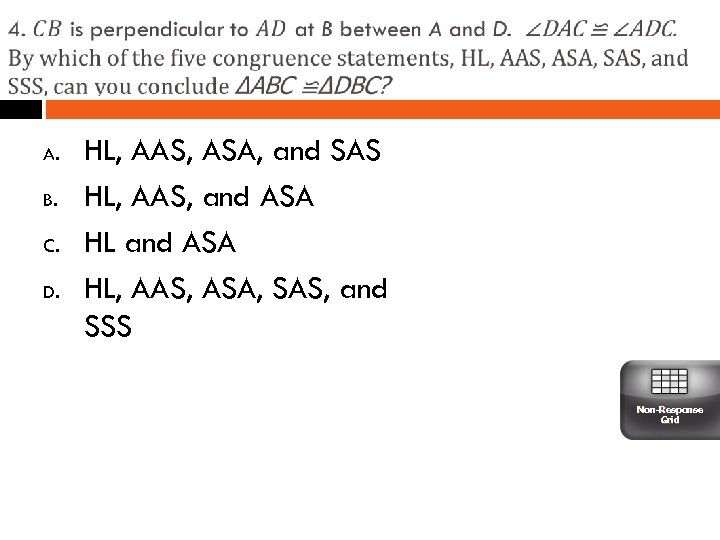A. B. C. D. HL, AAS, ASA, and SAS HL, AAS, and ASA HL, AAS, ASA, SAS, and SSS Non-Response Grid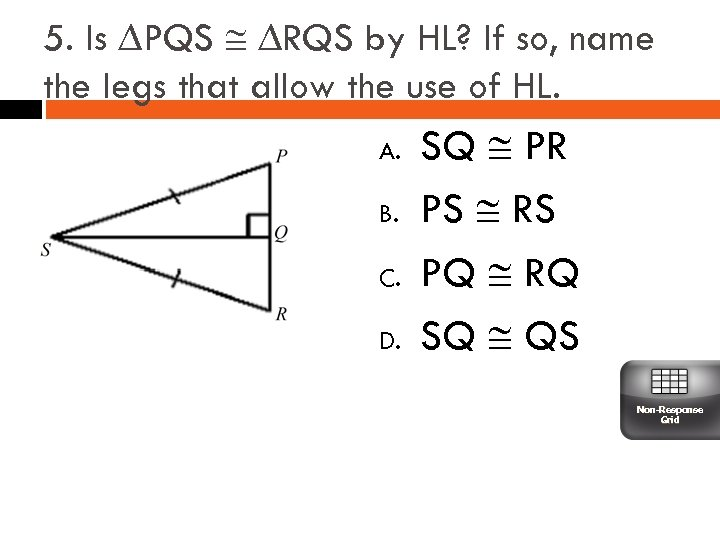5. Is ∆PQS ∆RQS by HL? If so, name the legs that allow the use of HL. A. B. C. D. SQ PR PS RS PQ RQ SQ QS Non-Response Grid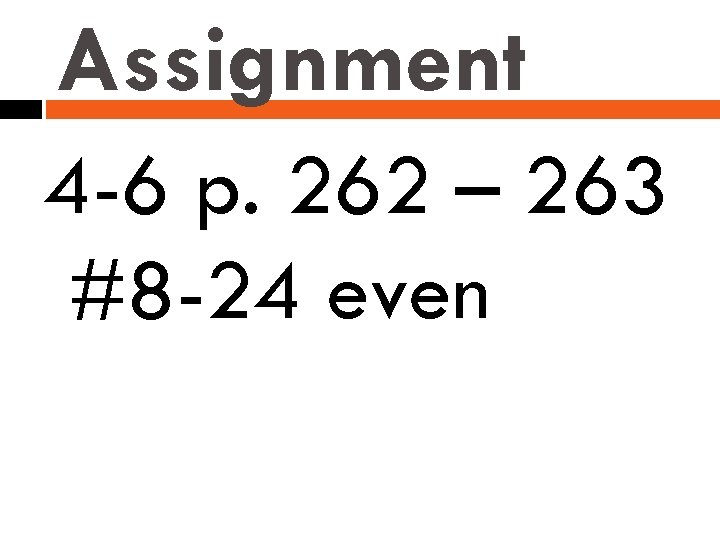Assignment 4 -6 p. 262 – 263 #8 -24 even